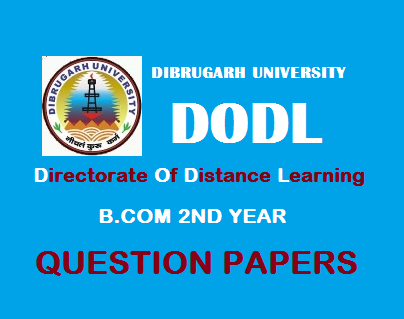# B.Com 2nd Year Question Papers (Distance): Indian Financial System' 20182018
(July)
COMMERCE
Paper: 206
(Indian Financial System)
Full Marks: 90
Time: 3 hours
The figures in the margin indicate full marks for the questions
1. Give direct answer: (any ten)                           1x10=10

1)         What do you mean by ‘Financial System’?
2)         Write one function of New Issue Market.
3)         What is ‘Scheduled Commercial Bank’?
4)         What do you mean by ‘Current Deposit’?
5)         Name the present Governor of Reserve Bank of India.
6)         What is ‘Demonetisation’?
7)         Write the full form of SEBI.
8)         Give the meaning of ‘SENSEX’.
9)         Write the full form of ‘OTCEI’.
10)      Write one objective of EXIM bank.
11)      Write one function of IDBI.
2. Write short notes on:                                  4x5=20
1)         Forex Market.
2)         Non-Performing Assets.
3)         Central Banking.
4)         Venture Capital Fund.
5)         Main objectives of NABARD.
3. (a) Discuss the Indian Financial System by highlighting its features and weaknesses.   6+6=12
Or
(b) What is ‘Money Market’? Mention the characteristics and functions of a money market.                      2+5+5=12
4. (a) Explain the nature and composition of assets maintained by commercial banks. What impact of asset structure of a bank has on its profitability?                   8+4=12
Or
(b) What is ‘Co-operative Banking’? Discuss the main objectives and functions of co-operative banking.        2+5+5=12
5. (a) Discuss the main functions of a Central Bank.                                          12
Or
(b) Discuss the objectives of Credit Control. Mention in details the credit control methods adopted by the Reserve Bank of India in Indian Banking System.                5+7=12
6. (a) What do you mean by Financial Market? Explain in brief its various types. Distinguish between Capital Market and Money Market.                                        2+4+6=12
Or
(b) What is ‘Stock Exchange’? Explain its significance in the development of Indian capital market.        2+10=12
7. (a) Discuss about the main objectives and functions of Industrial Development Bank of India.                              6+6=12
Or
(b) Explain the following:                                6+6=12
1)         State Financial Corporations.
2)         ICICI.